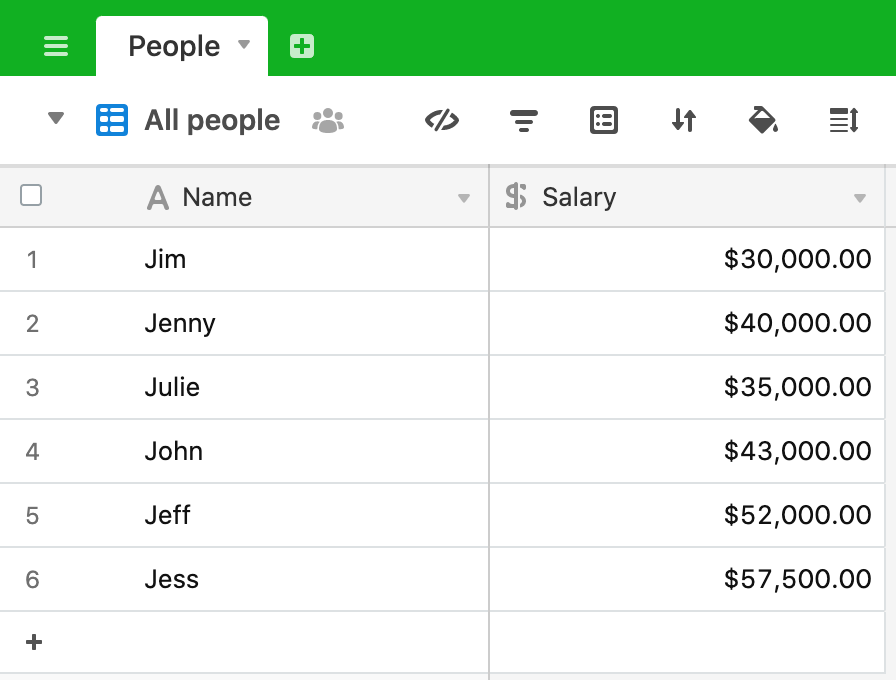# User input with buttons

In this post we’re going to write a script that gets some input from the user that changes what the script does - in this case, either proceed with an operation or cancel the operation.

Imagine this is your base, perhaps your HR base holding details of your employees and the salaries they earn.It is time for the annual review and your policy is to increase all salaries by the same rate (I’ve worked in a few companies where this always happens!). We could just update these one by one, but with a large team this will take a long time, so we’ve written a script that asks us by what percentage the salaries will increase, then applies this to the base.

Here’s the script:

``````
let table = base.getTable('People');
let query = await table.selectRecordsAsync();

let salaryIncrease = await input.textAsync('By what percentage would you like to increase the salaries?');
let cancelOrProceed = await input.buttonsAsync(`Increasing the salaries by \${salaryIncrease}%`, ['Cancel', 'Proceed']);

if (cancelOrProceed === 'Cancel') {
output.text('Salary increase cancelled');
} else {
output.text('Proceeding with salary increase');
for (let record of query.records) {
let newSalary = record.getCellValue('Salary') * (1 + Number(salaryIncrease)/100);
await table.updateRecordAsync(record, {
"Salary": newSalary
})
output.text(`Salary for \${record.name} updated`)
}
}

``````

Let’s break this down. We start by defining the table and querying it:

``````
let table = base.getTable('People');
let query = await table.selectRecordsAsync();

``````

We then ask the user to input the percentage they want to increase the salaries by:

``````
let salaryIncrease = await input.textAsync('By what percentage would you like to increase the salaries?');

``````

The user inputs a number and clicks on “Next”.

Our next line of code uses `input.buttonsAsync` to give the user a choice of responses:

``````
let cancelOrProceed = await input.buttonsAsync(`Increasing the salaries by \${salaryIncrease}%`, ['Cancel', 'Proceed']);

``````

In this case, we’re asking the user to respond by telling the script to proceed (and change all of the salaries) or cancel the operation (and leave the salaries as they are). The choice that the user makes (Cancel or Proceed) is assigned to the variable `cancelOrProceed` which we can now use in the remainder of the script. Two points to note here:

• The buttons and their labels are defined by the array at the end of the `input.buttonsAsync` method - `['Cancel', 'Proceed']`
• The values that the `cancelOrProceed` variable takes is the label text for each button
• You can have more than 2 buttons if your script needs them

We now come to an extremely common device in programming of any sort - the “if/else” statement. We’ve seen a simple “if” statement in a previous post. The “if/else” statement takes this a step further and has the general form:

``````
if (condition) {
//  code to be executed if the condition is true
} else {
//  code to be executed if the condition is false
}

``````

We use the “if/else” statement to decide which bit of code to run next. If the variable `cancelOrProceed` is equal to `Cancel` then we don’t want to update the salaries and we simply output some text to say that the operation has been cancelled. If the `cancelOrProceed` variable is equal to any other value then the “else” code will be executed - in this case, we’ll:

• Output some text so that the user knows what is happening
• Loop through all of the records from the query and update the salary by the percentage amount entered at the start.
• Let the user know when each record has been updated
``````
else {
output.text('Proceeding with salary increase');
for (let record of query.records) {
let newSalary = record.getCellValue('Salary') * (1 + Number(salaryIncrease)/100);
await table.updateRecordAsync(record, {
"Salary": newSalary
})
output.text(`Salary for \${record.name} updated`)
}
}

``````

In the “else” statement, we define a new variable `newSalary` and set this to be the current salary multiplied by `1 + % increase`. Note that when the user inputs the percentage increase value it is held as a string (even if they input a number), so we convert this to a number by using the `Number()` function.

## Recap

• Getting input from the user with the `input.textAsync` method
• Getting the user to choose between two code paths by providing buttons to click, using `input.buttonsAsync`
• Based on the choice made, cancelling the operation or proceeding to update the records